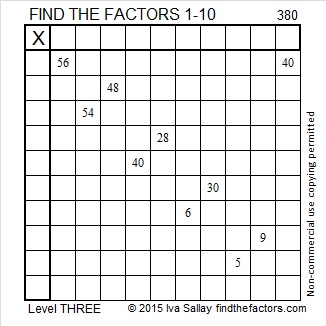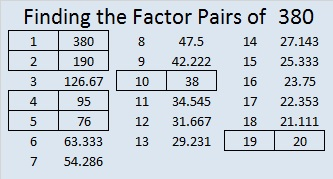# 380 and Level 3

380 is even so it has to be a composite number. Scroll down past the puzzle for all of its factors.Print the puzzles or type the factors on this excel file: 10 Factors 2015-02-02

• 380 is a composite number.
• Prime factorization: 380 = 2 x 2 x 5 x 19, which can be written 380 = (2^2) x 5 x 19
• The exponents in the prime factorization are 2, 1, and 1. Adding one to each and multiplying we get (2 + 1)(1 + 1)(1 + 1) = 3 x 2 x 2 = 12. Therefore 380 has exactly 12 factors.
• Factors of 380: 1, 2, 4, 5, 10, 19, 20, 38, 76, 95, 190, 380
• Factor pairs: 380 = 1 x 380, 2 x 190, 4 x 95, 5 x 76, 10 x 38, or 19 x 20
• Taking the factor pair with the largest square number factor, we get √380 = (√4)(√95) = 2√95 ≈ 19.494Since those consecutive numbers in the last factor pair are the last possibility on the chart, we know that 380 is the sum of the first 19 even numbers. Thus,

2 + 4 + 6 + 8 + 10 + 12 + 14 + 16 + 18 + 20 + 22 + 24 + 26 + 28 + 30 + 32 + 34 + 36 + 38 = 380. Those numbers can be rearranged this way:

2 + 36 + 4 + 34 + 6 + 32 + 8 + 30 + 10 + 28 + 12 + 26 + 14 + 24 + 16 + 22 + 18 + 20 + 38 = 380, which is more easily seen as the sum of ten 38’s.

A Logical Approach to FIND THE FACTORS: Find the column or row with two clues and find their common factor. Write the corresponding factors in the factor column (1st column) and factor row (top row).  Because this is a level three puzzle, you have now written a factor at the top of the factor column. Continue to work from the top of the factor column to the bottom, finding factors and filling in the factor column and the factor row one cell at a time as you go.

This site uses Akismet to reduce spam. Learn how your comment data is processed.